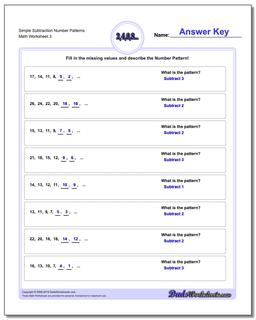# Math Worksheets: Number Patterns: Number Patterns: Simple Subtraction Number Patterns (Third Worksheet)## Simple Subtraction Number Patterns (Third Worksheet)

PropertyValue
DescriptionSimple Subtraction Number Patterns: Number pattern problems using only subtraction operations. (Third Worksheet)
Resource TypeWorksheet